# Water reservoir

The water reservoir is filled with one pump for 4 days by the second one for 9 days. Outlet can drain tank in 12 days. How long take fill the reservoir if both pumps are running and not closed outlet channel.

Result

t =  3.6

#### Solution:

t*(1/4+1/9-1/12)=1

10t = 36

t = 185 = 3.6

Calculated by our simple equation calculator.

Leave us a comment of example and its solution (i.e. if it is still somewhat unclear...):

Showing 0 comments:Be the first to comment!#### To solve this example are needed these knowledge from mathematics:

Need help calculate sum, simplify or multiply fractions? Try our fraction calculator. Do you have a linear equation or system of equations and looking for its solution? Or do you have quadratic equation?

## Next similar examples:

1. 12 Moons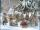Good Marry came to ask twelve moons for help with collecting strawberries. All twelve moons 1200 strawberries picked in 20 minutes. But lazy months July and August cease tear after 5 minutes. How many minutes in total will take collect strawberries?
2. Equation with fractionsSolve equation: ? It is equation with fractions.
3. Equation with xSolve the following equation: 2x- (8x + 1) - (x + 2) / 5 = 9
4. Pumps A and BPump A fill the tank for 12 minutes, pump B for 24 minutes. How long will take to fill the tank if he is only three minutes works A and then both pumps A and B?
5. Painters 4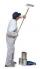One painter will do the job for 12 days. After a 4 1/3 day he comes second to help him and complete work together for 8 days. How long would work the second painter himself?
6. Construction of roadFor the construction of the road dam, it is supposed to transport six trucks a total of 18 days. After three days, two more cars were sent to the construction site. How long will it take to carry the material?
7. Bricklayers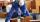8 bricklayers build a house for 630 days. How many bricklayers have to add after 150 days, so then the whole building completed in (next) 320 days?
8. Three peopleThree people start doing a work at a same time. The first worked only 2 hours. The second ended 3 hours before the end. On an individual basis, it would take the first time to do the work 10 hours, second 12 hours and 15 hours third. How many hours did it.
9. NovakNovak needed to dig up three of the same pit in the garden. The first pit dug father alone for 15 hours. His second dig son helped him and it did that in six hours. The third pit dug son himself. How long it took him?
10. Roses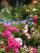On the large rosary was a third white, half red, yellow quarter and six pink. How many roses was in the rosary?
11. Unknown numberIdentify unknown number which 1/5 is 40 greater than one tenth of that number.
12. PearsThere were pears in the basket, I took two-fifths of them, and left six in the basket. How many pears did I take?
13. Simple equationSolve for x: 3(x + 2) = x - 18
14. EquationSolve the equation: 1/2-2/8 = 1/10; Write the result as a decimal number.
15. Dwarfs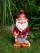Four dwarfs would prepare firewood for Snow White in580 minutes. After an hour and a half they recruit friends so that finished preparing the wood for 280 minutes. How many dwarfs they recruited?
16. BicycleThe bicycle pedal gear has 36 teeth, the rear gear wheel has 10 teeth. How many times turns rear wheel, when pedal wheel turns 120x?
17. Strawberries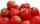Father collects strawberries himself in 4 hours, son in 14 hours. How long will it take them along when dad comes to help son collect strawberries after 3 hours?